# NCERT Solutions for Class 8 Maths Chapter 7 Cube and Cube Roots InText Questions

These NCERT Solutions for Class 8 Maths Chapter 7 Cube and Cube Roots InText Questions and Answers are prepared by our highly skilled subject experts.

## NCERT Solutions for Class 8 Maths Chapter 7 Cube and Cube Roots InText Questions

Try These (Page No. 111)

Question 1.
Find the one’s digit of the cube of each of the following numbers.
(i) 3331
(ii) 8888
(iii) 149
(iv) 1005
(v) 1024
(vi) 77
(vii) 5022
(viii) 53
Solution:
(i) 3331
unit digit of the number = 1
unit digit of the cube of the number = 1

(ii) 8888
unit digit of the number = 8
unit digit of the cube of the number = 2

(iii) 149
unit digit of the number = 9
unit digit of the cube of the number = 9(iv) 1005
unit digit of the number = 5
unit digit of the cube of the number = 5

(v) 1024
unit digit of the number = 4
unit digit of the cube of the number = 4

(vi) 77
unit digit of the number = 7
unit digit of the cube of the number = 3

(vii) 5022
unit digit of the number = 2
unit digit of the cube of the number = 8

(viii) 53
unit digit of the number = 3
unit digit of the cube of the number = 7Question 2.
Express the following numbers as the sum of odd numbers using the above pattern?
(a) 63
(b) 83
(c) 73
Solution:
(a) n = 6 and (n – 1) = 5
We have
63 = 31 + 33 + 35 + 37 + 39 + 41 = 216

(b) n = 8 and (n – 1) = 7
We have
83 = 57 + 59 + 61 + 63 + 65 + 67 + 69 + 71 = 512

(c) n = 7 and (n – 1) = 6
We have
73 = 43 + 45 + 47 + 49 + 51 + 53 + 53 + 55 = 343Question 3.
Consider the following pattern.
23 – 13 = 1 + 2 × 1 × 3
33 – 23 = 1 + 3 × 2 × 3
43 – 33 = 1 + 4 × 3 × 3
Using the above pattern, find the value of the following.
(i) 73 – 63
(ii) 123 – 113
(iii) 203 – 193
(iv) 513 – 503
Solution:
(i) 73 – 63 = 1 + 7 × 6 × 3 = 1 + 126 = 127
(ii) 123 – 113 = 1 + 12 × 11 × 3 = 1 + 396 = 397
(iii) 203 – 193 = 1 + 20 × 19 × 3 = 1 + 1140 = 1141
(iv) 513 – 503 = 1 + 51 × 50 × 3 = 1 + 7650 = 7651
Note: In the prime factorisation of any number, if each other appears three times, then the number is a perfect cube.

Try These (Page No. 112)

Question 4.
Which of the following are perfect cubes?
1. 400
2. 3375
3. 8000
4. 15625
5. 9000
6. 6859
7. 2025
8. 10648
Solution:
1. We have
400 = 2 × 2 × 2 × 2 × 5 × 5
2 × 5 × 5 remain after grouping in triples.
400 is not a perfect cube.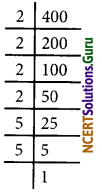2. We have
3375 = 3 × 3 × 3 × 5 × 5 × 5
The prime factors appear in triples.
3375 is a perfect cube.3. We have
8000 = 2 × 2 × 2 × 2 × 2 × 2 × 5 × 5 × 5
The prime factor of 8000 can be grouped into triples and no factor is left over.
8000 is a perfect cube.4. We have
15625 = 5 × 5 × 5 × 5 × 5 × 5
The Prime factors of 15625 can be grouped into triples and no factor is left over.
15625 is a perfect cube.5. We have
9000 = 2 × 2 × 2 × 3 × 3 × 5 × 5 × 5
The prime factors of 9000 cannot be grouped into tripes (because 3 × 3 are leftover).
9000 is not a perfect cube.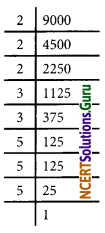6. We have
6859 = 19 × 19 × 19
The prime factors of 6859 can be grouped into triples and no factor is left over.
6859 is a perfect cube.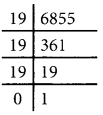7. We have
2025 = 3 × 3 × 3 × 3 × 5 × 5
We do not get triples of prime factors of 2025 and 3 × 5 × 5.
2025 is not a perfect cube.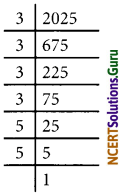8. We have
10648 = 2 × 2 × 2 × 11 × 11 × 11
The prime factor of 10648 can be grouped into triples and no factor is left over.
10648 is a perfect cube.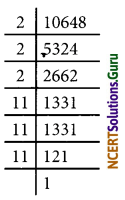error: Content is protected !!